IMO Shortlist 2010 problem A3

Kvaliteta:
Avg: 0,0
Težina:
Avg: 7,0
Let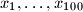$x_1, \ldots , x_{100}$ be nonnegative real numbers such that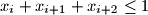$x_i + x_{i+1} + x_{i+2} \leq 1$ for all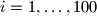$i = 1, \ldots , 100$ (we put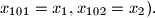$x_{101 } = x_1, x_{102} = x_2).$ Find the maximal possible value of the sum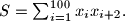$S = \sum^{100}_{i=1} x_i x_{i+2}.$

Proposed by Sergei Berlov, Ilya Bogdanov, Russia
Izvor: Međunarodna matematička olimpijada, shortlist 2010